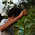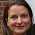## 05 April 2011

### How many bales do you need?

How many bales will you need?
To calculate the number of bales, I assume that we are building something without doors or windows.  That gives you enough extra bales for ample waste during installation, stuffing gaps, and straw for plastering as well.  You will want to know roughly the size of your bales.  Where I work on the East Coast, bales are usually 2-strings, usually about 14" high, 18" deep, and an average of 32" long (though length varies with any given stock of bales).  Once I have this information, I calculate the quantity of bales as follows:
1. Determine how many rows of bales high you will need for each wall height (not including any triangular gables).  For example, if your bales are 14" high, and your walls are 8-feet tall, then you will need 7 rows of bales for each wall.  (The number of rows will change if your walls are a different height, if your bales are a different dimension, or if you are laying the bales on edge.)
2. Calculate the total wall length for strawbale walls of that height.
3. Take your wall length in inches and divide by the average length of the bale.  For example, if I have a 10-foot long wall, that's 120-inches.  Assuming my bales are 32" long on average, that's 120 divided by 32, which equals 3.75 bales.  I round this up to the nearest 1/2-bale, in this case up to 4 bales.  This is the number of bales you need in each row.
4. Now multiply the number of bales in each row by the number of rows you need for your wall height.  In our example, this is 7 rows of bales with 4 bales in each row, or a total or 28 bales.
5. For gables, the calculation is number of rows needed at the peak of the gable, times number of bales needed along the first long row, divided by 2.
I use a little spreadsheet and calculate each wall separately.  Below is an example for a building with 8-foot tall walls, 2 peaked gable ends, and with exterior dimensions of 10-feet by 20-feet.

 Location Bales per row # of rows high Total Bales Level One East Wall 4 7 28 Level One West Wall 4 7 28 Level One South Wall (subtract width of East & West walls) 6.5 7 45.5 Level One North Wall (subtract width of East & West walls) 6.5 7 45.5 Level Two Gable Ends (2 gables so rows x bales only) 4 4 16 Total Bale Count 163

For a 10-foot x 20-foot building, the long walls are 240" and the short walls are 120".
The short East & West walls will be 120" divided by 32" (average bale length) = 3.75, so I round up to 4 bales per row.
The long South & North walls will be 240" minus the width of the corners (since the corners overlap) so 240" - 2(18" bale width) = 240" - 36" = 204".  Now divide by 32" (aver bale length) = 6.4, so I round up to 6.5 per row.
The height will be 8-feet tall, or 96".  Each bale is 14" tall, so 96" divided by 14" equals 6.8 bales tall, which we round up to 7 bales tall.
The gable dimensions vary depending on your roof slope and design, but notice my example shows 2 gable ends, so I do not need to divide each by 2 as you would if it were a single gable.

This will leave you with plenty of extra bales, assuming you have doors & windows in your building.  The extra bales allow you to reject any loose or poorly tied bales and give you plenty of straw to use for clay plasters, any cob walls, or an earthen floor.

#### 3 comments:

1.Hi again! How do I calculate the quantity of straw for cob mass? The same way as the strawbale?
I have a full identification in your work, thanks to the universe to meet you! =p

1.I'm so sorry to tell you, but I've never calculated the amount of straw I use in cob. Sorry!! Maybe make a small test wall and measure everything you use...then multiply to the size you are going to build

2.Thanks Sigi, I`ll gonna do that! I used this way to calculate the amount of clay plaster in a wall last month. =)